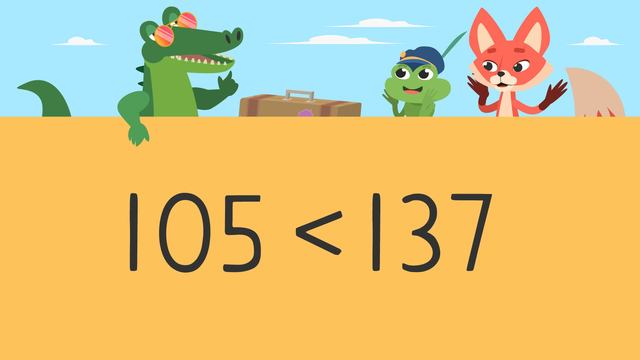# Compare 3 Digits (<, >, = )Rate this video

Ø 5.0 / 2 ratings

The authorTeam Digital
Compare 3 Digits (<, >, = )
CCSS.MATH.CONTENT.2.NBT.A.4

## Information about the videoCompare 3 Digits (<, >, = )

### In This Video

Dundee is visiting Skylar and Henry, and they are hungry. However, they will only eat food from the plate that has the most food on it, so Skylar and Henry will need to use comparing 3 digit numbers to decide which plate of food Dundee wants!

### How to Compare 3 Digit Numbers

To compare three-digit numbers, you start comparing the greatest place value you have, until you find a place value with a greater digit, otherwise you continue down the place value chart.

• If the hundreds place digits are the same, move to the tens place
• If the tens place digits are the same, move to the ones place
• Compare using appropriate symbol ( > < = )### Comparing 3 Digit Numbers 2nd Grade Example

When comparing 3 digit numbers, first set up a place value chart for each number, and place the numbers you are comparing inside them. Like in this one, we are comparing one hundred fifty-two with one hundred fifty-two.First, start in the hundreds place value and compare the numbers. We see one here in both places, so we can move to the tens place value because they are equal.Now we can compare the tens place value. We see five here in both places, so we can continue to the ones place value because they are equal.In the ones place value, we can see the number two in both places. This means they are equal.We have finished comparing, and we know every digit is equal to each other in each place value. This means that one hundred fifty-two is equal to one hundred fifty-two.### Comparing 3 Digit Numbers Summary

To compare three-digit numbers, you start in the highest place value. You compare each digit from left to right until you find a value that is greater. Then use the greater than, less than, or equal to signs to compare the numbers.

Below, you will find comparing 3-digit number worksheets for 2nd grade.

### TranscriptCompare 3 Digits (<, >, = )

Dundee is visiting Skylar and Henry and they are hungry. Dundee asks for food but will only eat food from whoever brings the most. Let's feed them by comparing three digit numbers. We can use the greater than, less than, or equal to symbols when comparing. A place value chart can help compare three digit numbers. We compare starting in the hundreds place, then the tens place, and finally the ones place. We stop comparing when we find a digit that is greater than or less than. If all place values are the same, the numbers are equal. For example, to compare these two numbers, start with the greatest place value, the hundreds. Check if the hundreds place digits are the same or different. There is one hundred in both, so move to the tens place. Check if the tens place digits are the same or different. There are five tens in both, so move to the ones place. Check if the ones place digits are the same or different. There are two ones, making these numbers equal, because all the place value digits are the same. One hundred fifty-two is equal to one hundred fifty-two. Oh, it looks like it's time to feed Dundee. Skylar gathered two hundred ten blueberries, and Henry gathered one hundred ninety-two. Starting in the greatest place value, we see two hundreds and one hundred. Two hundreds is greater than one hundred, so we do not need to continue comparing in the tens or ones places. Two hundred ten is greater than one hundred ninety-two. Dundee will guzzle up Skylar's plate of blueberries because it has more. Now Skylar and Henry have gathered grapes. Skylar gathered one hundred five grapes, and Henry gathered one hundred thirty-seven grapes. Start comparing in the hundreds place. Is one hundred greater than, less than, or equal to one hundred? One hundred is equal to one hundred, so move to the tens place and compare the digits. Are zero tens greater than, less than, or equal to three tens? Zero tens is less than three tens. We stop comparing here because we found a digit that is greater than or less than. Is one hundred five greater than, less than, or equal to one hundred thirty-seven? One hundred five is less than one hundred thirty-seven. Skylar's plate has less grapes, so Dundee devours Henry's plate of grapes. Before we see if Dundee is satisfied, let's review. Remember, when comparing three digit numbers, start with the greatest place value, the hundreds and compare. If the hundreds place digits are the same, move to the tens place and compare. If the tens place digits are the same, move to the ones place and compare. Finally, compare using the appropriate symbol, greater than, less than, or equal to. "Be very quiet Henry. We can't keep feeding them all our fruit!"